# 【Pytorch深度学习实战】（11）变分自动编码器（VAE）

🔎大家好，我是Sonhhxg_柒，希望你看完之后，能对你有所帮助，不足请指正！共同学习交流🔎

📝个人主页－Sonhhxg_柒的博客_CSDN博客 📃

🎁欢迎各位→点赞👍 + 收藏⭐️ + 留言📝​

📣系列专栏 – 机器学习【ML】 自然语言处理【NLP】  深度学习【DL】### 🖍foreword

✔说明⇢本人讲解主要包括Python、机器学习（ML）、深度学习（DL）、自然语言处理（NLP）等内容。

# Variational AutoEncoder（VAE）原理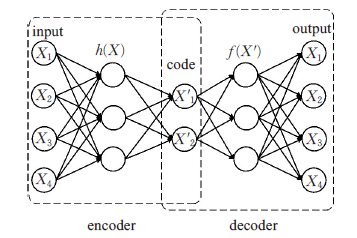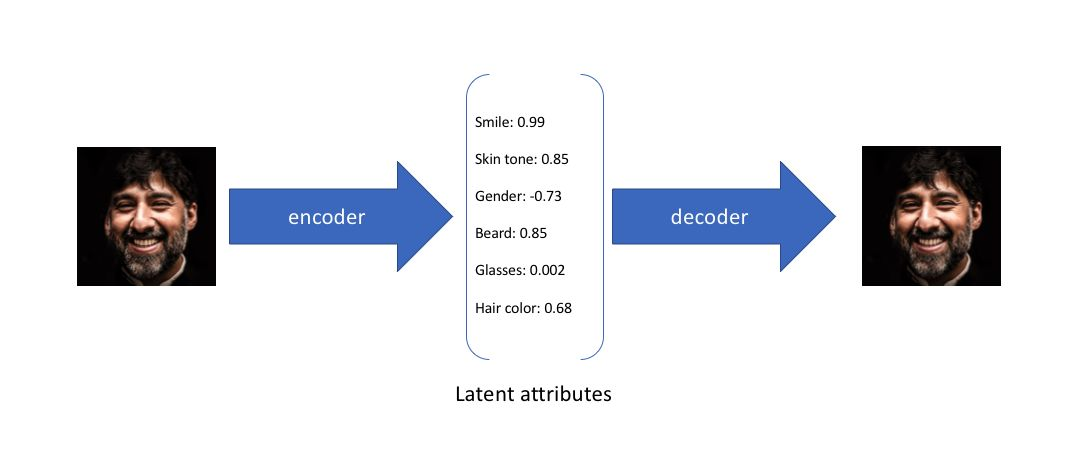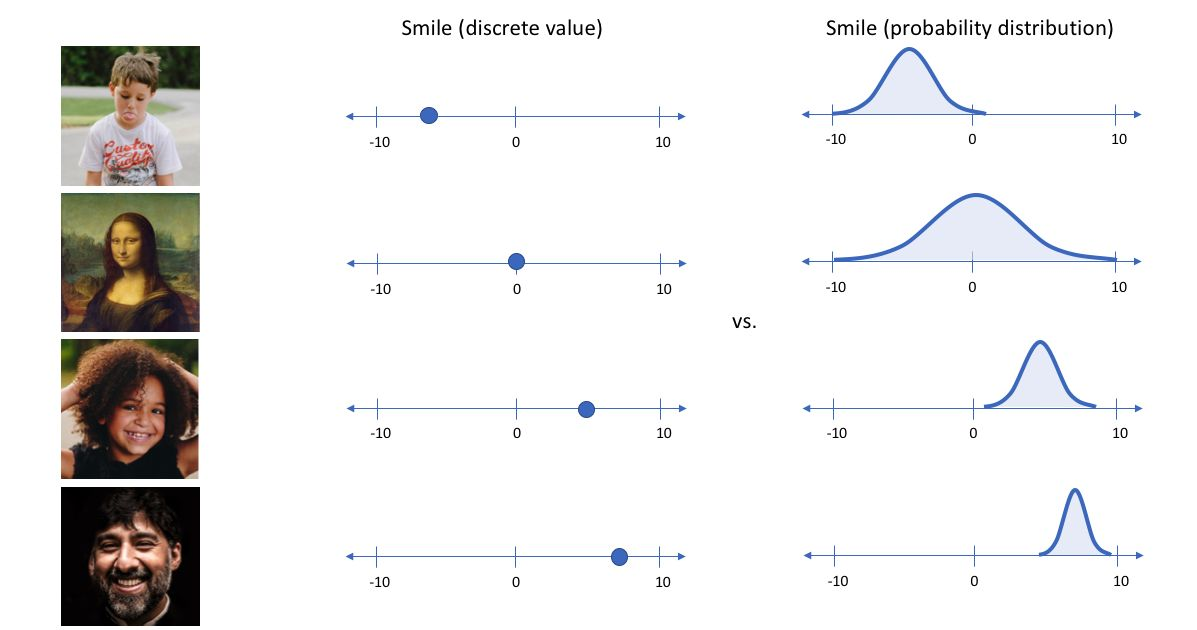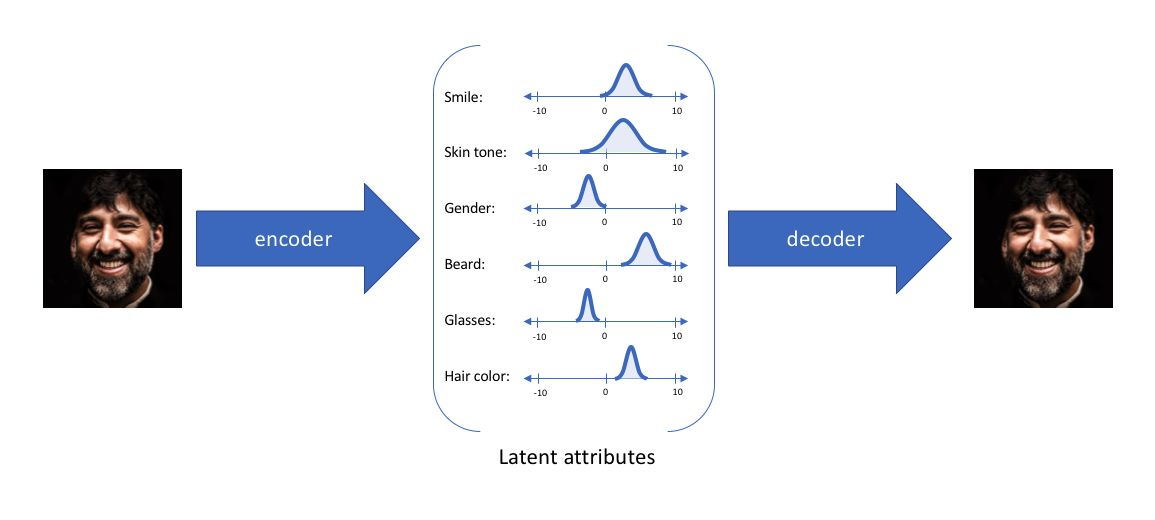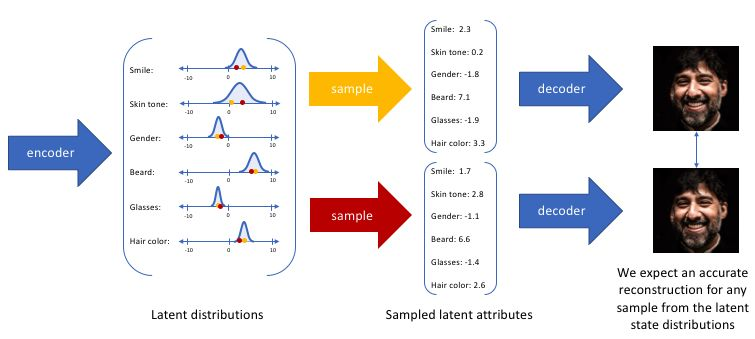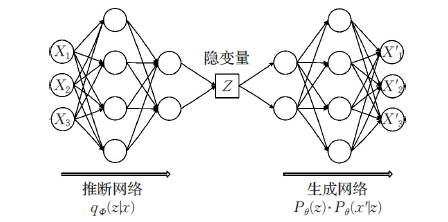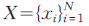，每个数据样本 xi 都是随机产生的相互独立、连续或离散的分布变量，生成数据集合为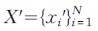，并且假设该过程产生隐变量Z ，即Z是决定X属性的神秘原因（特征）。其中可观测变量X 是一个高维空间的随机向量，不可观测变量 Z 是一个相对低维空间的随机向量，该生成模型可以分成两个过程：

（1）隐变量 Z 后验分布的近似推断过程：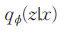，即推断网络。

（2）生成变量X' 的条件分布生成过程：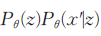，即生成网络。

## 变分自编码器Pytorch的实现

``````import os
import torch
import torch.nn as nn
import torch.nn.functional as F
import torchvision
from torchvision import transforms
from torchvision.utils import save_image

# 设备配置
device = torch.device('cuda' if torch.cuda.is_available() else 'cpu')

# 如果不存在则创建目录
sample_dir = 'samples'
if not os.path.exists(sample_dir):
os.makedirs(sample_dir)

# 超参数
image_size = 784
h_dim = 400
z_dim = 20
num_epochs = 15
batch_size = 128
learning_rate = 1e-3

# MNIST 数据集
dataset = torchvision.datasets.MNIST(root='../../data',
train=True,
transform=transforms.ToTensor(),

# 数据加载器
batch_size=batch_size,
shuffle=True)

# VAE模型
class VAE(nn.Module):
def __init__(self, image_size=784, h_dim=400, z_dim=20):
super(VAE, self).__init__()
self.fc1 = nn.Linear(image_size, h_dim)
self.fc2 = nn.Linear(h_dim, z_dim)
self.fc3 = nn.Linear(h_dim, z_dim)
self.fc4 = nn.Linear(z_dim, h_dim)
self.fc5 = nn.Linear(h_dim, image_size)

def encode(self, x):
h = F.relu(self.fc1(x))
return self.fc2(h), self.fc3(h)

def reparameterize(self, mu, log_var):
std = torch.exp(log_var/2)
eps = torch.randn_like(std)
return mu + eps * std

def decode(self, z):
h = F.relu(self.fc4(z))
return F.sigmoid(self.fc5(h))

def forward(self, x):
mu, log_var = self.encode(x)
z = self.reparameterize(mu, log_var)
x_reconst = self.decode(z)
return x_reconst, mu, log_var

model = VAE().to(device)

# 开始训练
for epoch in range(num_epochs):
for i, (x, _) in enumerate(data_loader):
# 前传
x = x.to(device).view(-1, image_size)
x_reconst, mu, log_var = model(x)

# 计算重建损失和kl散度
reconst_loss = F.binary_cross_entropy(x_reconst, x, size_average=False)
kl_div = - 0.5 * torch.sum(1 + log_var - mu.pow(2) - log_var.exp())

# 反向传播和优化
loss = reconst_loss + kl_div
loss.backward()
optimizer.step()

if (i+1) % 10 == 0:
print ("Epoch[{}/{}], Step [{}/{}], Reconst Loss: {:.4f}, KL Div: {:.4f}"
.format(epoch+1, num_epochs, i+1, len(data_loader), reconst_loss.item(), kl_div.item()))

# 保存采样图像
z = torch.randn(batch_size, z_dim).to(device)
out = model.decode(z).view(-1, 1, 28, 28)
save_image(out, os.path.join(sample_dir, 'sampled-{}.png'.format(epoch+1)))

# 保存重建的图像
out, _, _ = model(x)
x_concat = torch.cat([x.view(-1, 1, 28, 28), out.view(-1, 1, 28, 28)], dim=3)
save_image(x_concat, os.path.join(sample_dir, 'reconst-{}.png'.format(epoch+1)))``````

(0)